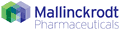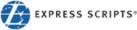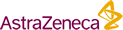#### Get the Book: Make Better Decisions

Finding and Evaluating Generic and Branded Drug Market Entry Opportunities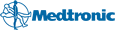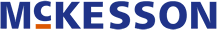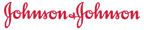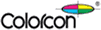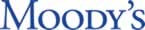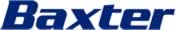Last Updated: December 12, 2019

DrugPatentWatch Database Preview

# Claims for Patent: 5,952,375

Get access to a free drug patent landscape report or a free one-month subscription

« Back to Dashboard

##### Summary for Patent: 5,952,375
 Title: Compounds and methods for synthesis and therapy Abstract: Novel compounds are described. The compounds generally comprise an acidic group, a basic group, a substituted amino or N-acyl and a group having an optionally hydroxylated alkane moiety. Pharmaceutical compositions comprising the inhibitors of the invention are also described. Methods of inhibiting neuraminidase in samples suspected of containing neuraminidase are also described. Antigenic materials, polymers, antibodies, conjugates of the compounds of the invention with labels, and assay methods for detecting neuraminidase activity are also described. Inventor(s): Bischofberger; Norbert W. (San Carlos, CA), Kim; Choung U. (San Carlos, CA), Lew; Willard (San Mateo, CA), Liu; Hongtao (Foster City, CA), Williams; Matthew A. (Foster City, CA) Assignee: Gilead Sciences, Inc. (Foster City, CA) Application Number: 08/606,624 Patent Litigation and PTAB cases: See patent lawsuits and PTAB cases for patent 5,952,375 Patent Claims: 1. A composition comprising a compound of the formula: ##STR461## wherein: E.sub.1 is --CO.sub.2 H, --CO.sub.2 R.sub.5, --CO.sub.2 R.sub.5a W.sub.5 or --CO.sub.2 W.sub.5 ; G.sub.1 is --N(R.sub.11).sub.2, --N(R.sub.11)C(N(R.sub.11))(N(R.sub.11).sub.2), or --C(R.sub.11).sub.2 --N(R.sub.11).sub.2 ; T.sub.1 is --NH(C(O)CH.sub.3), --NH(C(O)CH.sub.2 F), --NH(C(O)CHF.sub.2), or --NH(C(O)CF.sub.3); U.sub.1 is --OR.sub.4, --SR.sub.4, NHR.sub.4 or N(R.sub.4).sub.2 ; R.sub.1 is independently H or alkyl of 1 to 12 carbon atoms; R.sub.2 is independently R.sub.3 or R.sub.4 wherein each R.sub.4 is independently substituted with 0 to 3 R.sub.3 groups; R.sub.3 is independently F, Cl, Br, I, --CN, N.sub.3, --NO.sub.2, --OR.sub.6a, --OR.sub.1, --N(R.sub.1).sub.2, --N(R.sub.1)(R.sub.6b), --N(R.sub.6b).sub.2, --SR.sub.1, --SR.sub.6a, --S(O)R.sub.1, --S(O).sub.2 R.sub.1, --S(O)OR.sub.1, --S(O)OR.sub.6a, --S(O).sub.2 OR.sub.1, --S(O).sub.2 OR.sub.6a, --C(O)OR.sub.1, --C(O)R.sub.6c, --C(O)OR.sub.6a, --OC(O)R.sub.1, --N(R.sub.1)(C(O)R.sub.1), --N(R.sub.6b)(C(O)R.sub.1), --N(R.sub.1)(C(O)OR.sub.1), --N(R.sub.6b)(C(O)OR.sub.1), --C(O)N(R.sub.1).sub.2, --C(O)N(R.sub.6b)(R.sub.1), --C(O)N(R.sub.6b).sub.2, --C(NR.sub.1)(N(R.sub.1).sub.2), --C(N(R.sub.6b))(N(R.sub.1).sub.2), --C(N(R.sub.1))(N(R.sub.1)(R.sub.6b)), --C(N(R.sub.6b))(N(R.sub.1)(R.sub.6b)), --C(N(R.sub.1))(N(R.sub.6b).sub.2), --C(N(R.sub.6b))(N(R.sub.6b).sub.2), --N(R.sub.1)C(N(R.sub.1))(N(R.sub.1).sub.2), --N(R.sub.1)C(N(R.sub.1))(N(R.sub.1)(R.sub.6b)), --N(R.sub.1)C(N(R.sub.6b))(N(R.sub.1).sub.2), --N(R.sub.6b)C(N(R.sub.1))(N(R.sub.1).sub.2), --N(R.sub.6b)C(N(R.sub.6b))(N(R.sub.1).sub.2), --N(R.sub.6b)C(N(R.sub.1))(N(R.sub.1)(R.sub.6b)), --N(R.sub.1)C(N(R.sub.6b))(N(R.sub.1)(R.sub.6b), --N(R.sub.1)C(N(R.sub.1))(N(R.sub.6b).sub.2), --N(R.sub.6b)C(N(R.sub.6b))(N(R.sub.1)R.sub.6b)), --N(R.sub.6b)C(N(R.sub.1))(N(R.sub.6b).sub.2), --N(R.sub.1)C(N(R.sub.6b))(N(R.sub.6b).sub.2), --N(R.sub.6b)C(N(R.sub.6b))(N(R.sub.6b).sub.2), .dbd.O, .dbd.S, .dbd.N(R.sub.1) or .dbd.N(R.sub.6b); R.sub.4 is independently alkyl of 1 to 12 carbon atoms, alkenyl of 2 to 12 carbon atoms, or alkynyl of 2 to 12 carbon atoms; and R.sub.5 is independently R.sub.4 wherein each R.sub.4 is substituted with 0 to 3 R.sub.3 groups; R.sub.5a is independently alkylene of 1 to 12 carbon atoms, alkenylene of 2 to 12 carbon atoms, or alkynylene of 2-12 carbon atoms any one of which alkylene, alkenylene or alkynylene is substituted with 0-3 R.sub.3 groups; R.sub.6a is independently H or an ether- or ester-forming group; R.sub.6b is independently H, a protecting group for amino or the residue of a carboxyl-containing compound; R.sub.6c is independently H or the residue of an amino-containing compound; W.sub.5 is carbocycle or heterocycle wherein W.sub.5 is independently substituted with 0 to 3 R.sub.2 groups; and R.sub.11 is independently H or R.sub.5. 2. The composition of claim 1 wherein: G.sub.1 is --NH.sub.2, --N(H)C(N(H))(NH.sub.2), --NHCH.sub.3, --NHCH.sub.2 CH.sub.3, --N(CH.sub.3).sub.2, --N(CH.sub.2 CH.sub.3).sub.2, --N(CH.sub.3)(CH.sub.2 CH.sub.3), --NHCH.sub.2 CH.sub.2 OH, --NHCH.sub.2 CH.sub.2 NH.sub.2, or --CH.sub.2 --NH.sub.2 ; T.sub.1 is --N(H)(C(O)CH.sub.3), --N(H)(C(O)CH.sub.2 F), --N(H)(C(O)CHF.sub.2), or --N(H)(C(O)CF.sub.3); and R.sub.4 is --OR.sub.4, --SR.sub.4 or NHR.sub.4 is branched chain. 3. The composition of claim 1 wherein R.sub.4 is alkyl of 1 to 12 carbon atoms, and R.sub.11 is R.sub.4. 4. The composition of claim 1 wherein R.sub.4 is alkyl of 1 to 8 carbon atoms, or alkenyl or alkynyl of 2 to 8 carbon atoms, and R.sub.11 is R.sub.4. 5. The composition of claim 1 wherein R.sub.4 is alkyl of 1 to 6 carbon atoms, or alkenyl or alkynyl of 2 to 6 carbon atoms, and R.sub.11 is R.sub.4. 6. The composition of claim 1 wherein R.sub.4 is alkyl of 1 to 6 carbon atoms, and R.sub.11 is R.sub.4. 7. The composition of claim 1 wherein R.sub.4 is alkyl of 1 to 6 carbon atoms and each R.sub.11 is H. 8. The composition of claim 1 wherein E.sub.1 is C(O)OCH.sub.2 CH.sub.3 ; G.sub.1 is NH.sub.2, NHCH.sub.3 or NHCH.sub.2 CH.sub.3 ; T.sub.1 is NH(C(O)CH.sub.3); and U.sub.1 is OCH(CH.sub.2 CH.sub.3).sub.2.

### Make Better Decisions: Try a trial or see plans & pricing